# C++ Pointer

### What is a pointer?

It’s a representation of addresses which is an indicator that points to an address of a value.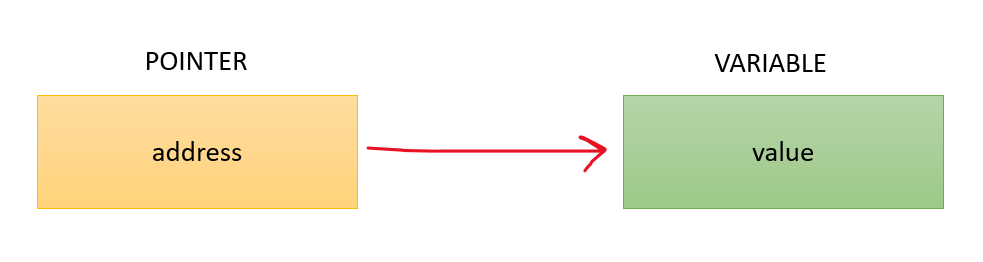Syntax:

``` datatype*var_name ;
float*ptr  ;  // ptr point to address which holds float data
```

Working of Pointer: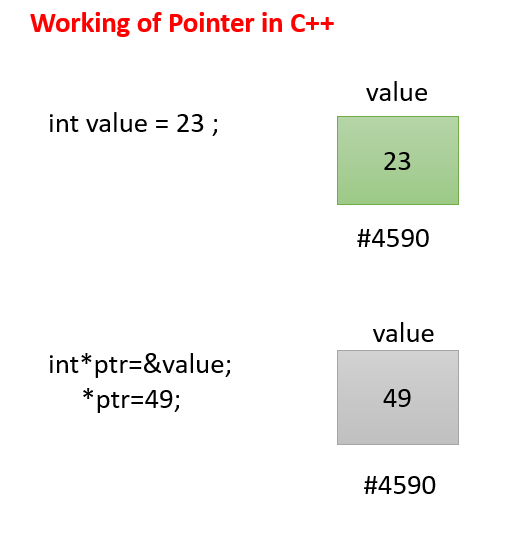• Unary Operator (&) assigns the address of a variable to the pointer. This returns the address of the variable.
• Unary Operator(*) is used to access the value stored in the address which returns the value of the variable.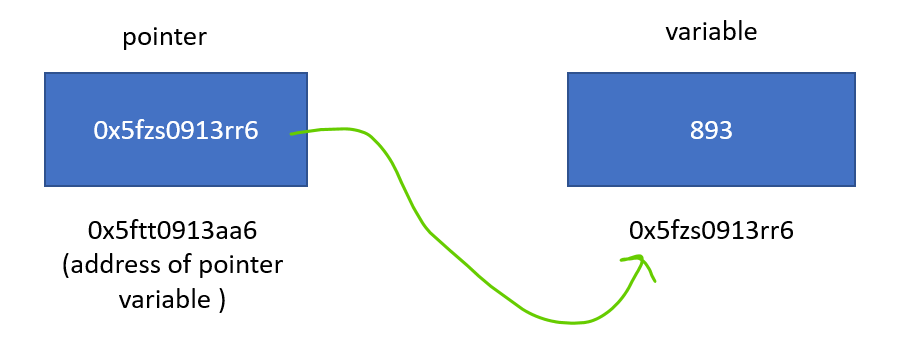0x at the beginning shows the address in Hexadecimal form.

Code:

## C++ Code

``````#include <bits/stdc++.h>

using namespace std;
int main() {

int val = 433;

int * ptr; // pointer variable

ptr = & val; // assigning address of val to ptr

cout << "Value of val : " << val << "\n";
cout << "Value of pointer ptr and Address of val :  " << ptr << "\n";
cout << "Value of *ptr : " << * ptr << "\n";
return 0;
}``````

Output:

Value of val : 433
Value of pointer ptr and Address of val : 0x7fe0c95a5b9c
Value of *ptr : 433

### Difference between Reference and Pointers

Pointer: A pointer is a variable that holds the memory address of another variable.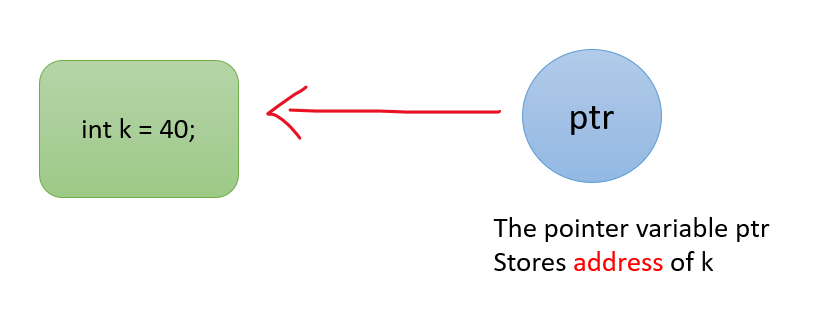• It can also be assigned to point a NULL value
• A pointer can be changed to point to any variable of the same data type.
• The pointer needs to be dereferenced with a *.

Reference: It is a variable that is referred to another name for an already existing variable.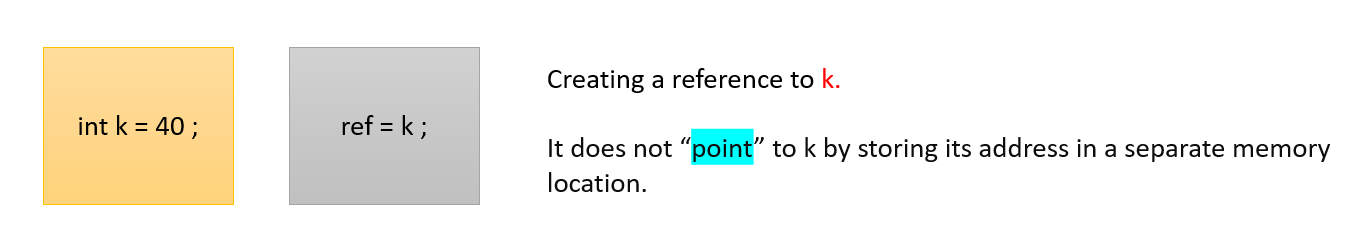• Reference cannot be a NULL value
• Reference cannot be changed to refer to a variable object.

### Array Name as Pointers

• The array name is used as a pointer to store elements in the array
• After that, we print the elements of the array using the same pointer. The compiler creates a pointer by default while we create an array.
• For example, if we have an array as arr, then arr and & arr both can be used.

Code:

## C++ Code

``````#include<bits/stdc++.h>

using namespace std;

int main() {

int arr = {1,2,3,4};

int * ptr;
ptr = & arr;

cout << "Values of Array\n";
cout << ptr << " " << ptr << " " << ptr << " " << ptr << "\n";
cout << "Address of each element of Array\n";
cout << & ptr << " " << & ptr << " " << & ptr << " " << & ptr << "\n";
return 0;
}``````

Output:

Values of Array
1 2 3 4
Address of each element of Array
0x7f54117f5b80 0x7f54117f5b84 0x7f54117f5b88 0x7f54117f5b8c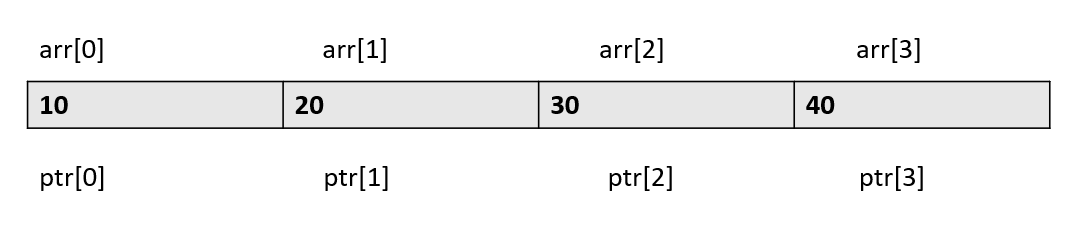### Pointer Arithmetic

• The following operations can be performed on the Pointers: Addition and Subtraction
• An integer can be added to a pointer
• An integer can be subtracted from a pointer
• The difference can also be taken from 2 pointers

Code:

## C++ Code

``````#include <bits/stdc++.h>

using namespace std;
int main() {

int arr = {10,20,30,40,50};

int * ptr;

ptr = & arr;

/*Traversing from left to right using addition */
cout << "Left to Right\n";
for (int i = 0; i < 5; i++) {
cout << "Value of pointer ptr: " << ptr << "\n";
cout << "Value of pointer *ptr: " << * ptr << "\n";

ptr += 1;
}
cout << "\n\n";

cout << "Right to Left\n";
ptr = & arr;
/*Traversing from right to left using addition */
for (int i = 4; i >= 0; i--) {
cout << "Value of pointer ptr: " << ptr << "\n";
cout << "Value of pointer *ptr: " << * ptr << "\n";

ptr -= 1;
}

return 0;
}``````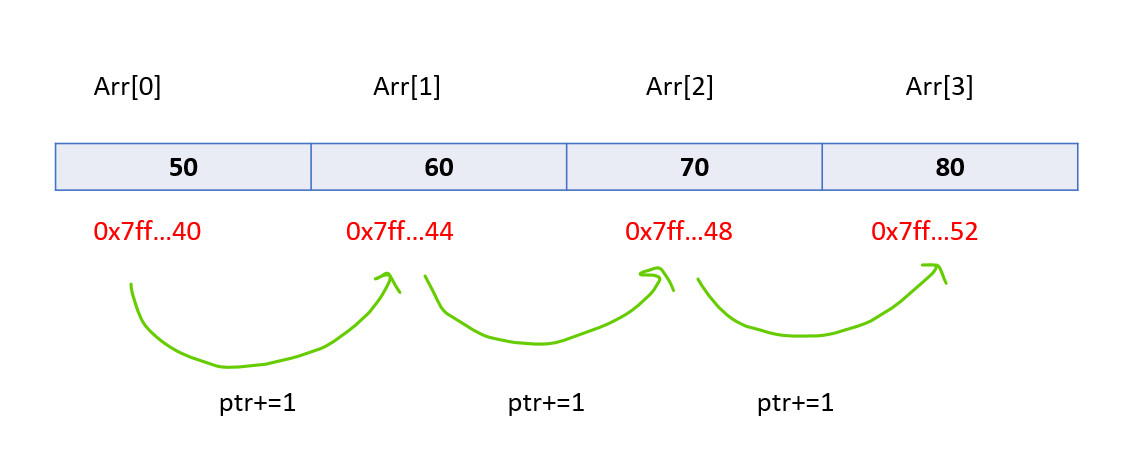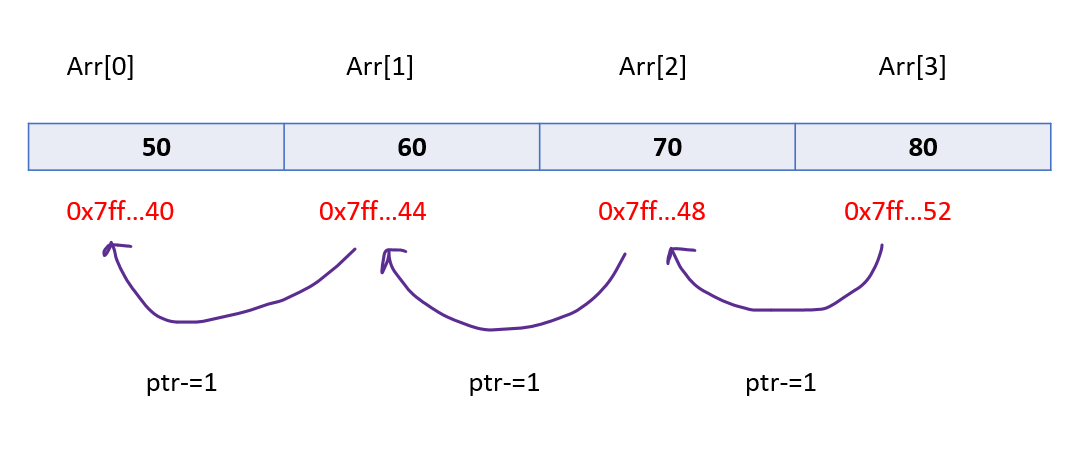Special thanks to Shreyas Vishwakarma for contributing to this article on takeUforward. If you also wish to share your knowledge with the takeUforward fam, please check out this article